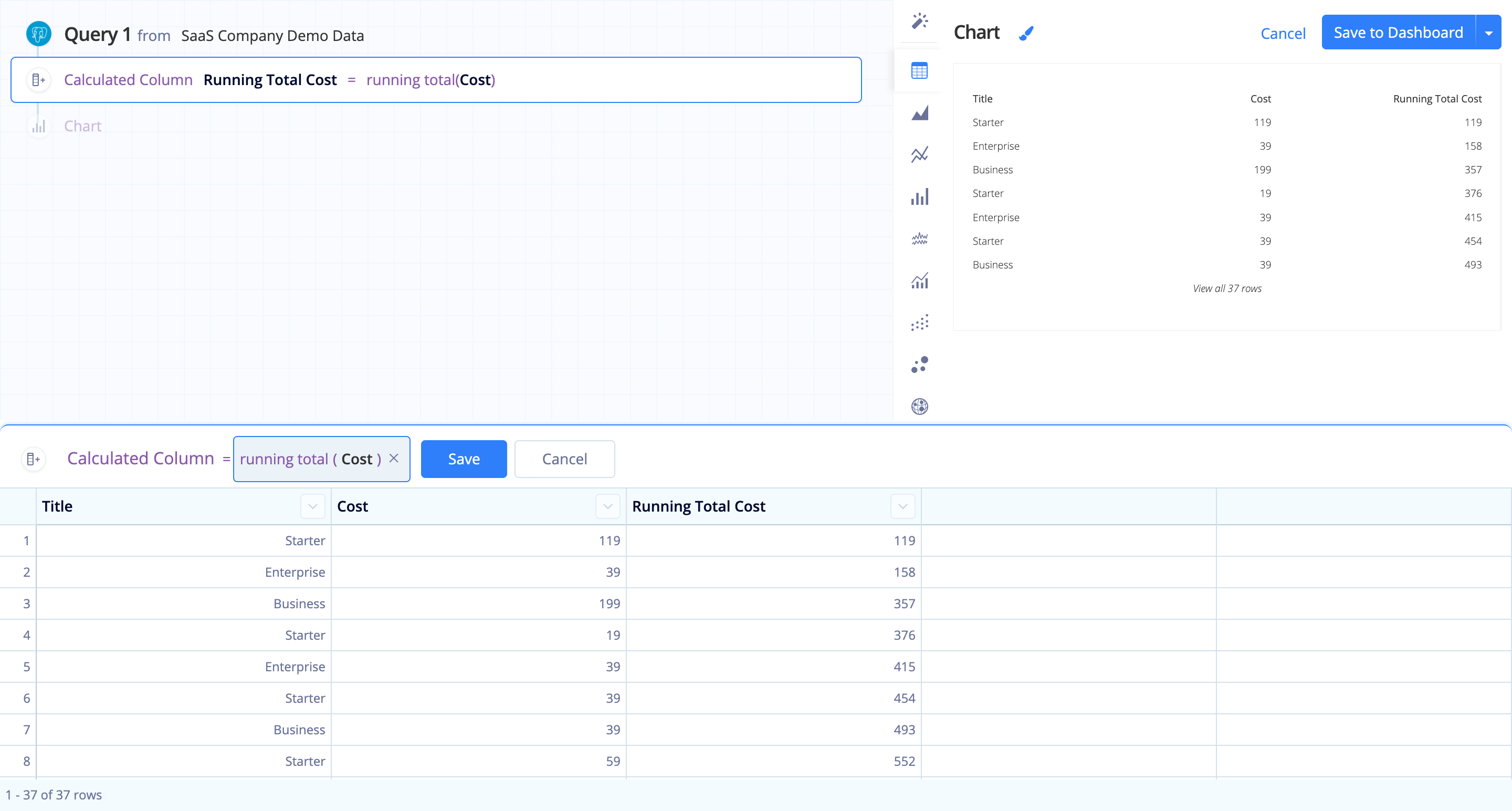# Guided Formulas

When selecting a Guided Formula, you’re prompted to select values for each required field. There’s also additional text to help you decide which column to choose or what value to input for each field. No need to worry about the syntax–we handle it for you.

Note: If you aren’t seeing a formula type you expect to see for an existing column, check the column’s data type. Some formulas are only available for numeric columns.

Single Row Operations Multi Row Operations
Column Ratio Lag
Create Link with Title Moving Average
Date Difference Percent Change
Divide Percentile
Extract Text Ratio of Total
Format Running Total
Multiply
Round
Subtract
Total Column Sum

## Single row operations

Add a numerical value to a column. If you want to add a column to another column, you’ll need to use a Custom formula.

### Column Ratio

Easily divide one column by another in your result set to get a ratio. Integers are auto-converted to decimals to ensure decimal accuracy.

First choose a column for the numerator, then choose a column for the denominator.

Use a text column and a URL column and generate a Markdown link that’s clickable from your Table chart, using the text column as the link title.

Useful for creating short, easily parsable links.

### Date Difference

Calculate the amount of time between two dates. Choose which time unit the value is calculated in: days, weeks, months, etc.

### Divide

Divide a column by a set value. If you want to divide a column by another column, you’ll need to use a Custom formula.

### Extract Text

Select a specific part of a string, where that substring is added to a new column. Choose the starting index (1 being the first letter), then specify a length or leave it empty to include all characters to the end of the string.

If your start and end positions vary, check out our FAQ for using Custom Formulas to extract text.

### Format

Specify decimal precision (i.e., number of decimal places), useful for force-formatting currency. Format returns a string, not an integer. Therefore, it is best used in table charts or Single Value charts.

### Multiply

Multiply a column by a value. If you want to multiply a column by another column, you’ll need to use a Custom formula.

### Round

Round is used to round to a specific number of decimal places.

Note: You cannot round a number to a higher decimal precision than it already has. For example, you cannot round the integer 10 to have two decimal places.

### Subtract

Subtract a value from a column. If you want to subtract a column from another column, you’ll need to use a Custom formula.

### Total Column Sum

Create a new column where each value is a sum of the values in its row.

## Multi row operations

### Aggregation

Create a new column with an aggregation value of another column. Choose from AVERAGE, COUNT, MAX, MEDIAN, MIN, or SUM.

Useful for applying calculations to a column that involve an aggregated value. For example, use the SUM aggregation then add another Formula Column and use Column Ratio to determine a column’s value percentage of the total column.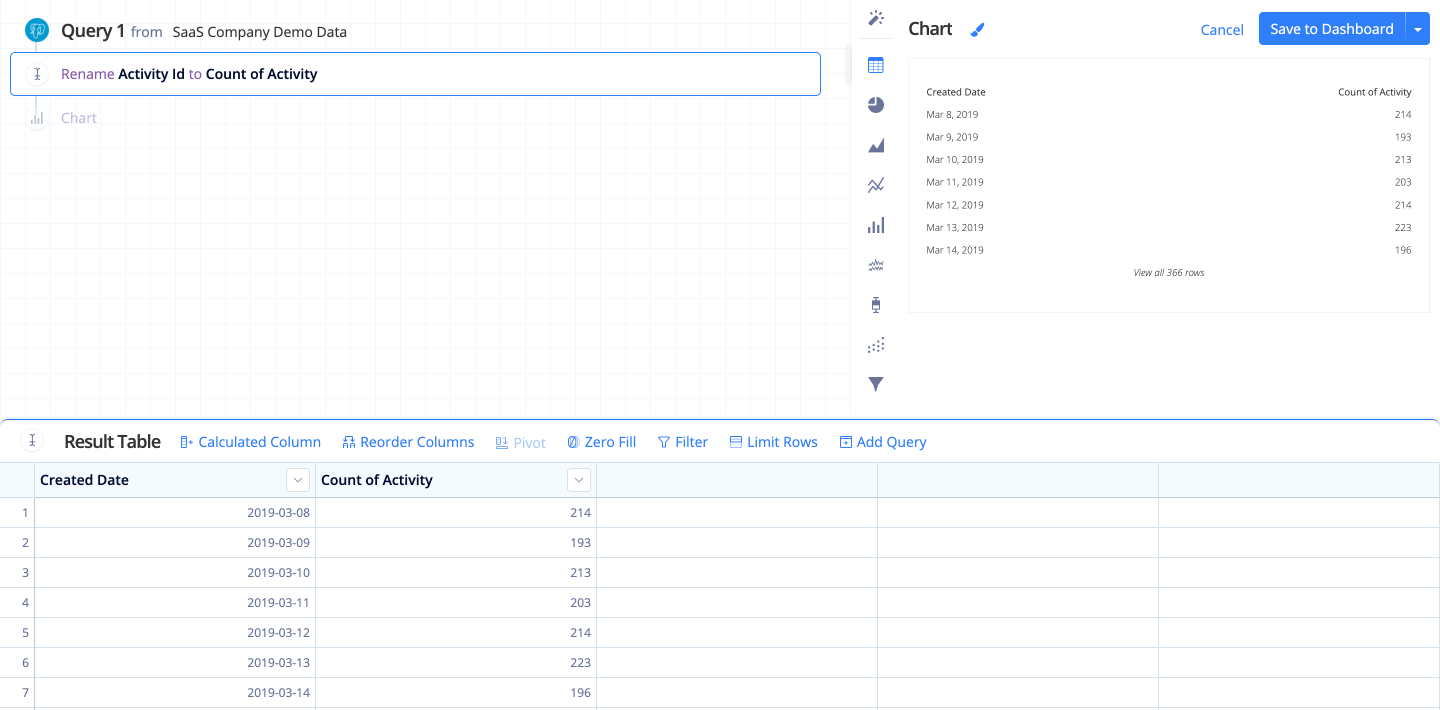### Lag

Access data from a previous row in an existing column. Row offset defines the number of rows back from the current row to use.

Useful for comparing the value of a row with the value of the previous row.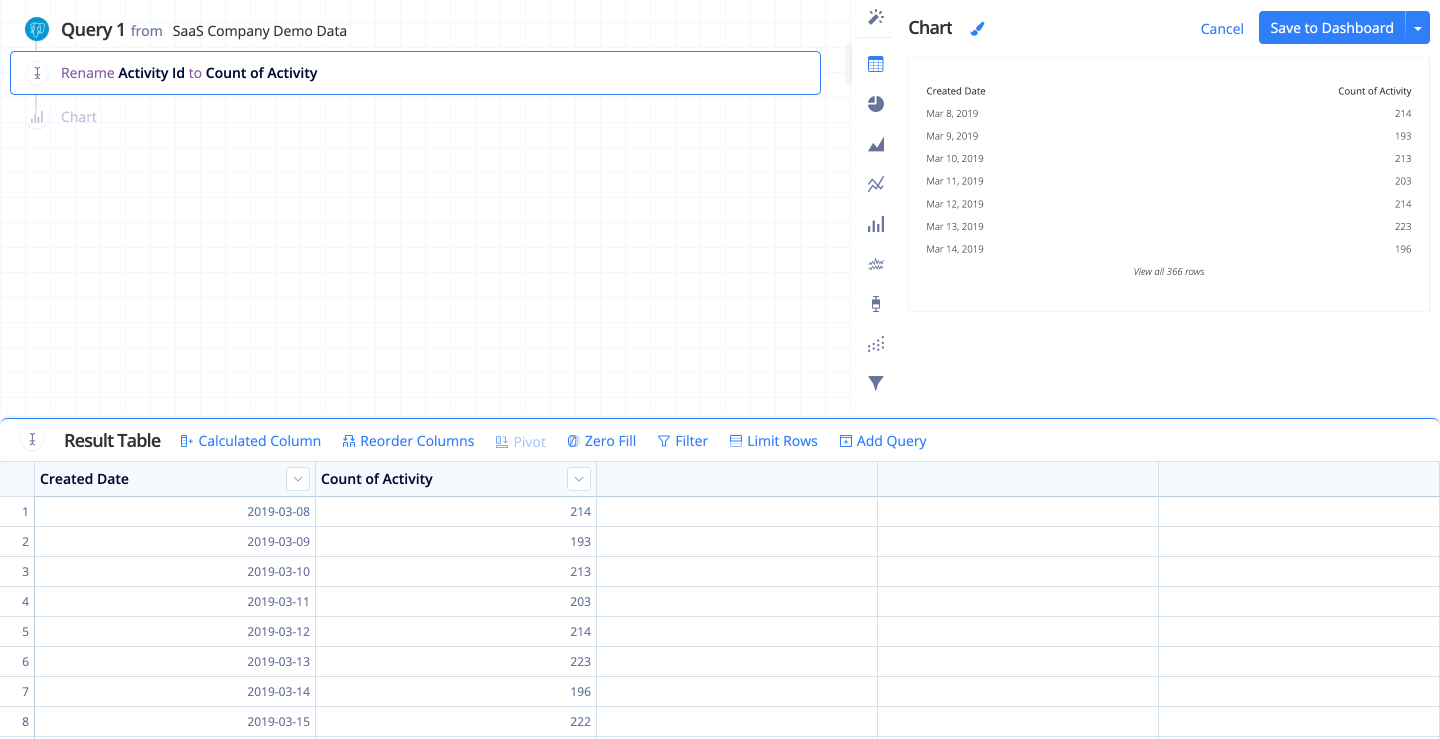### Moving Average

Generate a series of averages of your data to create a smooth trend line. Trailing rows is the number of previous rows to include in each row’s average.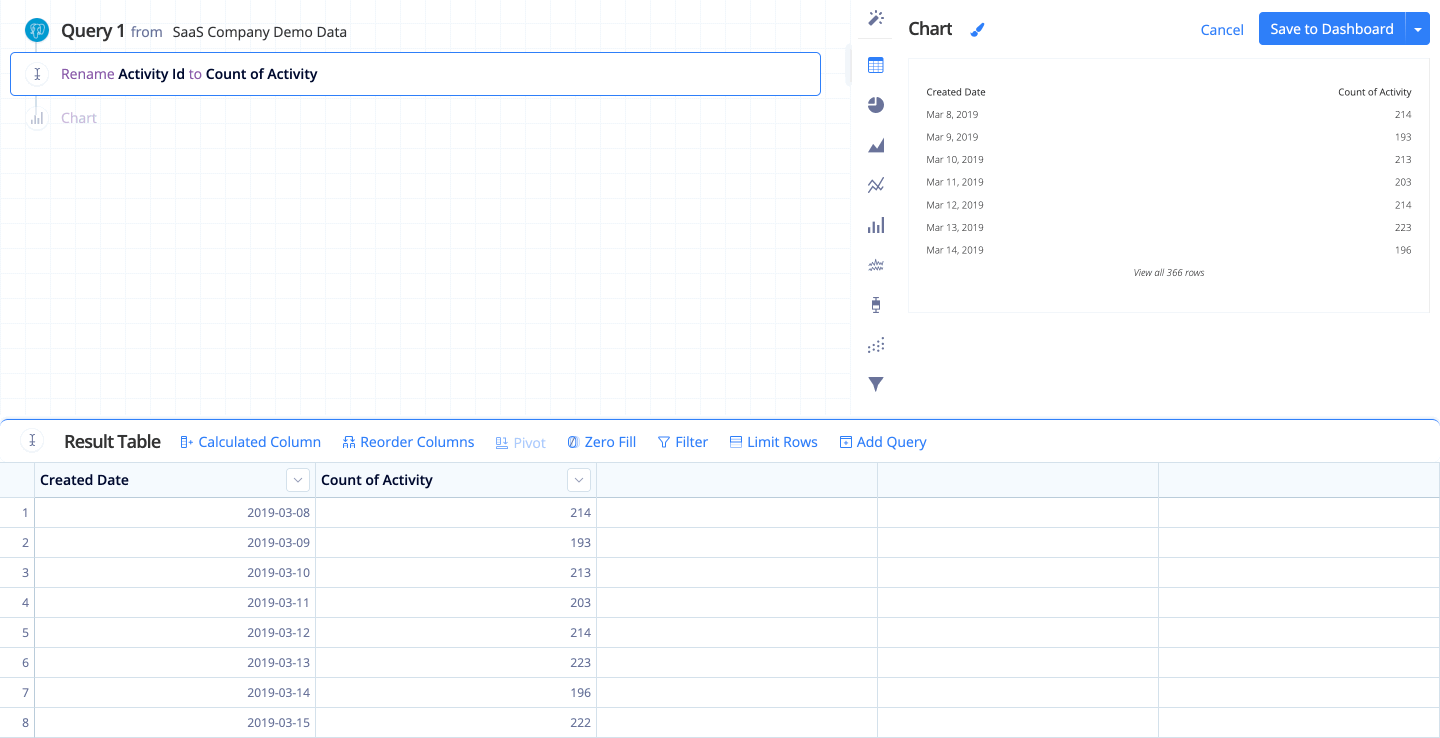### Percent Change

Determines the percent change between the current and previous row.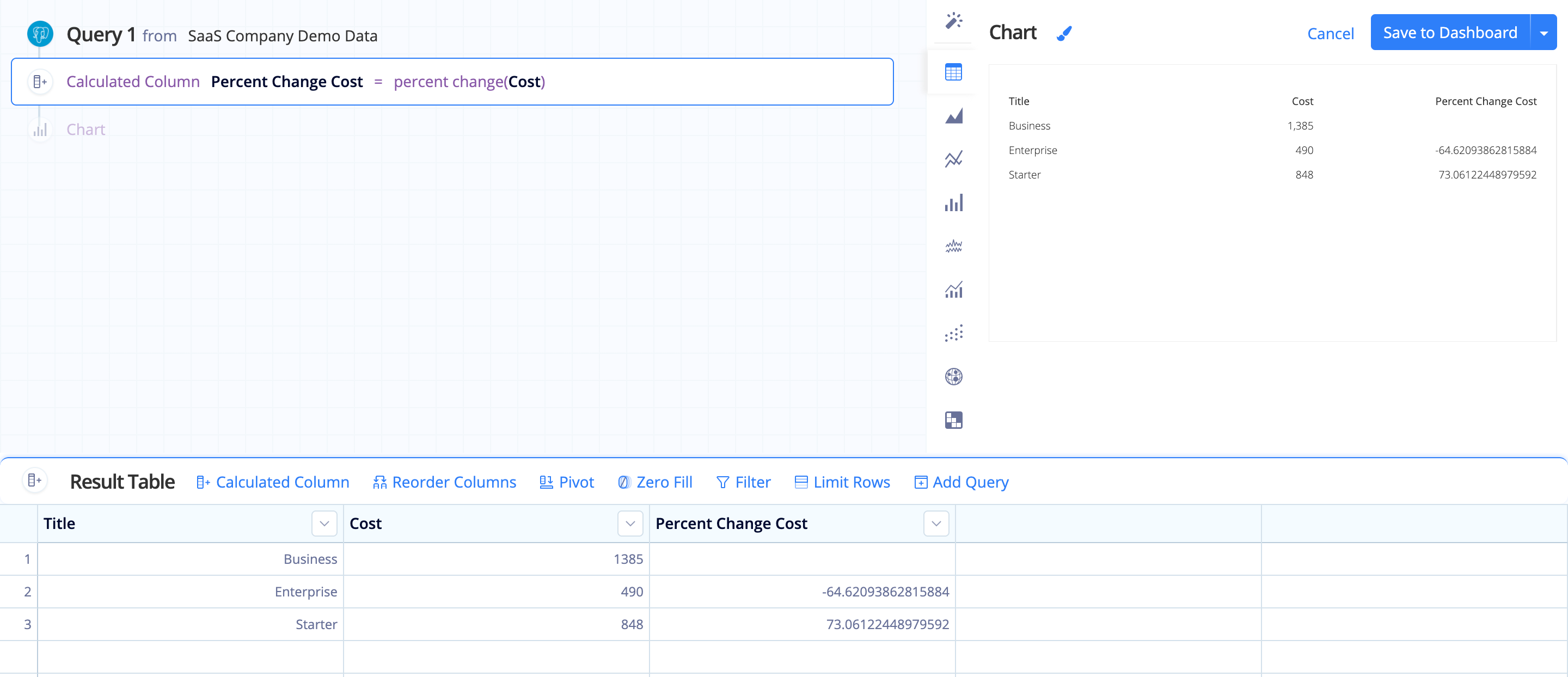### Percentile

Determines the percentile of each value in a numeric column. For example, a value is at the 50th percentile if half of the values in the column are less than or equal to it.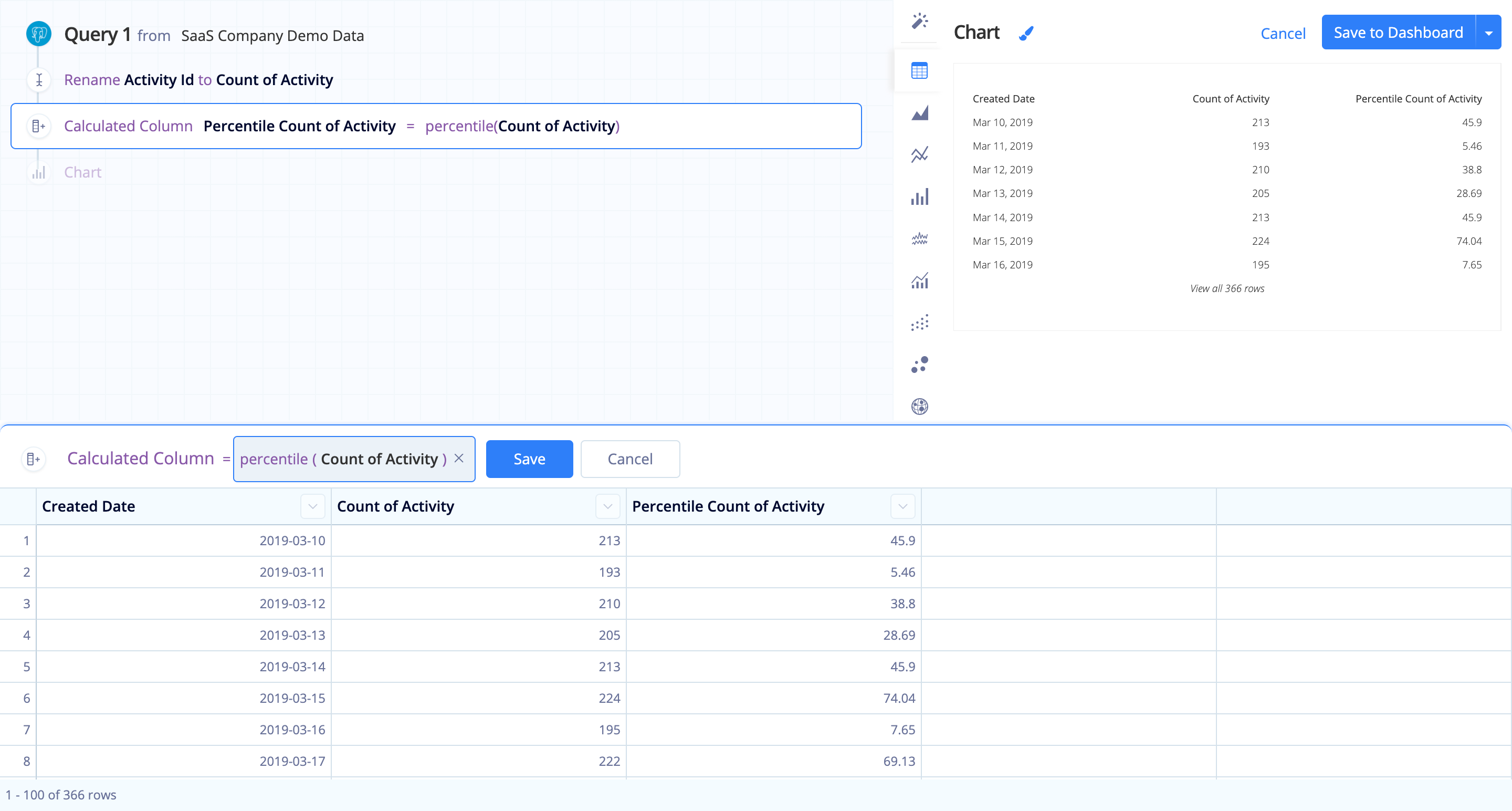### Ratio of Total

Displays each row’s value as a ratio of the column sum (i.e., the row’s value divided by the column’s total sum).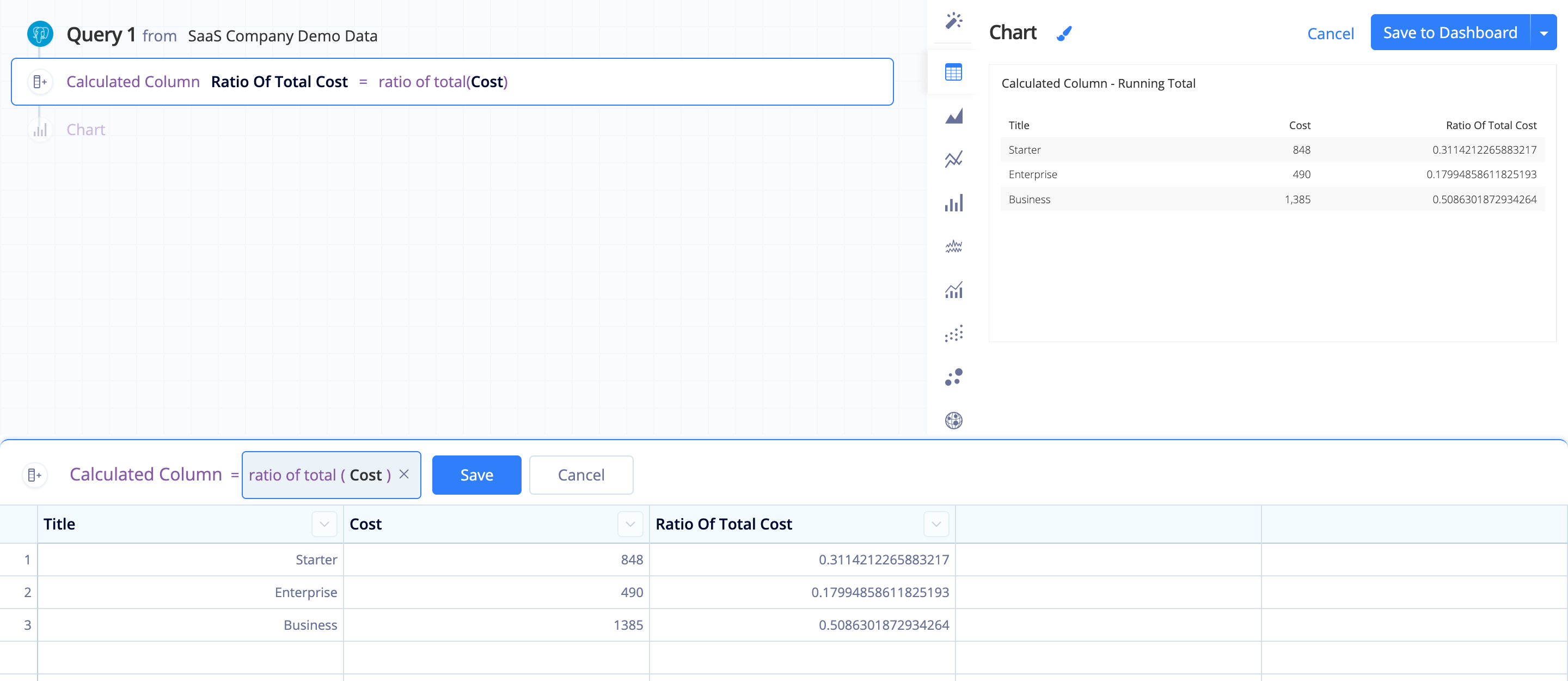### Running Total

Cumulative sum of all previous results of a column (i.e., row1, row1+row2, row1+row2+row3, etc.).